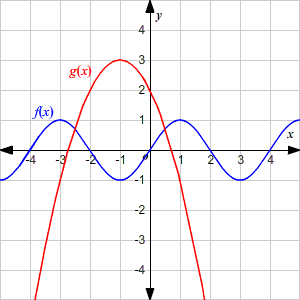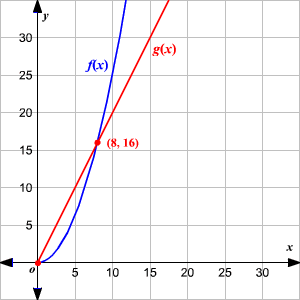# Describing the Graph of a Function

Sometimes you need to describe the graph of a function in a non-symbolic way. For example, you may be asked

1. whether a function is increasing or decreasing;

2. whether it has one minimum value or maximum value, or several such values

3. whether it is linear or not

4. whether the rate of change is constant, increasing, or decreasing

5. whether it has an upper or lower bound.

Example 1:

Describe the two functions $f\left(x\right)$ and $g\left(x\right)$ , using the terms increasing, decreasing, maxima and minima.The graph of $f\left(x\right)$ is periodic. It decreases for $-3 , then increases for $-1 , then decreases again for $1 , etc.

It has a maximum value of $1$ and a minimum value of $-1$ , and it attains these maxima and minima many times. The upper bound of the function is $1$ and the lower bound is $-1$ .

The graph of $g\left(x\right)$ is increasing for $-\infty and decreasing for $-1 . The graph takes a maximum value of $3$ at $x=-1$ . It has no minimum.

Example 2:

Which graph has a greater rate of change?Both graphs start together at $\left(0,0\right)$ . At first, the linear function, $g\left(x\right)$ , has the faster rate of change.

But $f\left(x\right)$ soon catches up, and surpasses $g\left(x\right)$ at $\left(8,16\right)$ , and continues increasing at a faster rate.